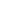Primary Maths Tutoring - St George & Sutherland Community College
Results for »
Primary Maths Tutoring

# Primary Maths Tutoring

Whether your child needs help with basic maths, filling in the gaps where their understanding of the concept may be shaky, or are preparing for advance maths in OC classes, Selective High or Extension classes our maths tutors can help your child reach their academic goals.

X

## Maths Tutoring - Year 2

This practical course is designed to teach the student to master Stage 1 Mathematical concepts. This program integrates the components of working mathematically through whole number, addition and subtraction, multiplication and division, fractions and decimals, patterns and

...
[More]
\$225 Limited GST free
Maths Tutoring - Year 2

<p>This practical course is designed to teach the student to master Stage 1 Mathematical concepts. This program integrates the components of working mathematically through whole number, addition and

...
Mo Tu We Th Fr Sa Su\$225 Limited GST free
Maths Tutoring - Year 2

<p>This practical course is designed to teach the student to master Stage 1 Mathematical concepts. This program integrates the components of working mathematically through whole number, addition and

...
Mo Tu We Th Fr Sa Su## Maths Tutoring - Year 3

This practical course will teach the student effective strategies and develop their conceptual understanding of Early Stage 2 Math. The students Math coaching is individualised to cater for the educational needs of the students who wish to understand the key concepts in Math or

...
[More]
\$225 Limited GST free
Maths Tutoring - Year 3

<p>This practical course will teach the student effective strategies and develop their conceptual understanding of Early Stage 2 Math. The students

Math coaching is individualised to cater for the

...
Mo Tu We Th Fr Sa Su\$225 Limited GST free
Maths Tutoring - Year 3

<p>This practical course will teach the student effective strategies and develop their conceptual understanding of Early Stage 2 Math. The students

Math coaching is individualised to cater for the

...
Mo Tu We Th Fr Sa Su## Maths Tutoring - Year 4

This practical course will teach the student effective strategies and develop their conceptual understanding of Stage 2 Maths concepts. This program integrates the components of working mathematically through whole number, addition and subtraction, multiplication and division,

...
[More]
\$225 Limited GST free
Maths Tutoring - Year 4

<p>This practical course will teach the student effective strategies and develop their conceptual understanding of Stage 2 Maths concepts. This program integrates the components of working

...
Mo Tu We Th Fr Sa Su\$225 Limited GST free
Maths Tutoring - Year 4

<p>This practical course will teach the student effective strategies and develop their conceptual understanding of Stage 2 Maths concepts. This program integrates the components of working

...
Mo Tu We Th Fr Sa Su## Maths Tutoring - Year 5

This practical course will teach the student effective strategies and develop their conceptual understanding of Early Stage 3 Maths concepts.This program integrates the components of working mathematically through whole number, addition and subtraction, multiplication and

...
[More]
\$225 Limited GST free
Maths Tutoring - Year 5

<p>This practical course will teach the student effective strategies and develop their conceptual understanding of Early Stage 3 Maths concepts.This program integrates the components of working

...
Mo Tu We Th Fr Sa Su\$225 Limited GST free
Maths Tutoring - Year 5

<p>This practical course will teach the student effective strategies and develop their conceptual understanding of Early Stage 3 Maths concepts.This program integrates the components of working

...
Mo Tu We Th Fr Sa Su## Maths Tutoring - Year 6

This practical course will teach the student effective strategies and develop their conceptual understanding of Stage 3 Maths concepts. This program integrates the components of working mathematically through whole number, addition and subtraction, multiplication and division,

...
[More]
\$225 Limited GST free
Maths Tutoring - Year 6

<p>This practical course will teach the student effective strategies and develop their conceptual understanding of Stage 3 Maths concepts. This program integrates the components of working

...
Mo Tu We Th Fr Sa Su\$225 Limited GST free
Maths Tutoring - Year 6

<p>This practical course will teach the student effective strategies and develop their conceptual understanding of Stage 3 Maths concepts. This program integrates the components of working

...
Mo Tu We Th Fr Sa Su## Maths Tutoring - Year 1

This practical course is designed to teach the student to master Early Stage 1 Mathematical concepts.This program integrates the components of working mathematically through whole number, addition and subtraction, multiplication and division, fractions and decimals, patterns and

...
[More]
\$225 Limited GST free
Maths Tutoring - Year 1

<p>This practical course is designed to teach the student to master Early Stage 1 Mathematical concepts.This program integrates the components of working mathematically through whole number, addition

...
Mo Tu We Th Fr Sa Su## Whole Number - Stage 1 Master Class - Year 2

These practical master classes are designed to teach the student Stage 1 Mathematical concepts. This program integrates the components of working mathematically through whole number, addition and subtraction, multiplication and division. Professional qualified and experienced

...
[More]

[More]

## Whole Number - Stage 2 Master Class - Years 3 and 4

These practical master classes are designed to teach the student Stage 2 Mathematical concepts. This program integrates the components of working mathematically through whole number, addition and subtraction, multiplication and division. Professional qualified and experienced

...
[More]

## Fractions, Decimals & Percentages - Stage 2 Master Class - Years 3 and 4

These practical master classes are designed to teach the student effective strategies and develop their conceptual understanding in Fractions, Decimals and Percentages. Professional qualified and experienced teachers help your child realise their full potential through sequential

...
[More]